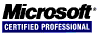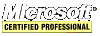# PHP Tips and Tricks

## Databases

### DBM Databases

DBM databases are simple, efficient flat files that limit the structure of the data to key/value pairs. To know which DBM handlers are installed use `dba_handlers`. DB2, DB3, DB4, DBM, GDBM, NDBM, QDBM, CDB are examples.

``````<?php
print '<pre>';
print_r(dba_handlers());
print '</pre>';
?>
``````

This code resulted in this output:

```Array
(
 => cdb
 => cdb_make
 => db4
 => inifile
 => flatfile
)
```

#### Using DBM Databases

To get a handle to a DBM flat file, use `dba_open`. It takes three arguments. The first is the DBM file. The second is file open mode. A value of `r` is for read-only access, while `w` is for opening an existing database with read-write access. A value of `c` opens a database for read-write access and will create the database file if it does not exist. Finally, `n` does the same thing as `c` but truncates the file. The third argument is which DBM handler to use.

``````<?php
\$dbh = dba_open(__DIR__.'/colors.db','c','db4') or die(\$php_errormsg);
?>
``````

Use `dba_exists` to determine if a key exists in the file. Use `dba_fetch` to get the value. Use `dba_replace` to replace a value. Use `dba_delete` to delete a key/value.

``````<?php
\$key = \$_POST['color_key'];
\$new_value = \$_POST['color_value'];
if(dba_exists(\$key, \$dbh)) {
\$value = dba_fetch(\$key, \$dbh);
if(\$value != \$new_value) {
dba_replace(\$key, \$new_value, \$dbh);
}
} else {
\$delete = false;
if(\$delete) {
dba_delete(\$key, \$dbh);
} else {
dba_insert(\$key, \$new_value, \$dbh);
}
}
?>
``````

To loop through all the key/value pairs, use `dba_firstkey`, `dba_nextkey` and `dba_fetch`.

``````<?php
for(\$key = dba_firstkey(\$dbh); \$key !== false; \$key = dba_nextkey(\$dbh)) {
\$value = dba_fetch(\$key, \$dbh);
}
?>
``````

Finally, when done with the database file, close it with `dba_close`.

``````<?php
dba_close(\$dbh);
?>
``````

A full example:

``````<html>
<body>
<form method="post" action="<?php echo \$_SERVER['SCRIPT_NAME'] ?>">
<select name="color_key">
<?php
\$dbh = dba_open(__DIR__.'/colors.db','c','db4') or die(\$php_errormsg);
if(\$_SERVER['REQUEST_METHOD'] == 'POST') {
\$key = \$_POST['color_key'];
\$new_value = \$_POST['new_color'];
if(dba_exists(\$key, \$dbh)) {
\$value = dba_fetch(\$key, \$dbh);
if(\$value != \$new_value) {
dba_replace(\$key, \$new_value, \$dbh);
}
} else {
dba_insert(\$key, \$new_value, \$dbh);
}
}
for(\$key = dba_firstkey(\$dbh); \$key !== false; \$key = dba_nextkey(\$dbh)) {
print '<option value="'.\$key.'">'.dba_fetch(\$key, \$dbh).'</option>';
}
dba_close(\$dbh);
?>
</select>
<input type="text" name="new_color" placeholder="enter a new name" />
<input type="submit" value="change this color" />
</form>
<hr />
<form method="post" action="<?php echo \$_SERVER['SCRIPT_NAME'] ?>">
<input type="hidden" name="color_key" value="<?php echo uniqid() ?>" />
<input type="text" name="new_color" placeholder="enter a new color" />
<input type="submit" value="add this color" />
</form>
</body>
</html>
``````

### SQL Databases

SQL databases are usually relational and this makes them very powerful. To use an SQL database with PHP, it must be told to include support for that database when compiled unless PHP supports dynamic module loading.

PHP's PDO database access layer allows the programmer to use the same PHP functions no matter what database is being used.

#### MySQL Databases

This section under construction.

#### ODBC

This section under construction. However, PHP can support the ODBC API.

<< < [Page 4 of 6] > >>